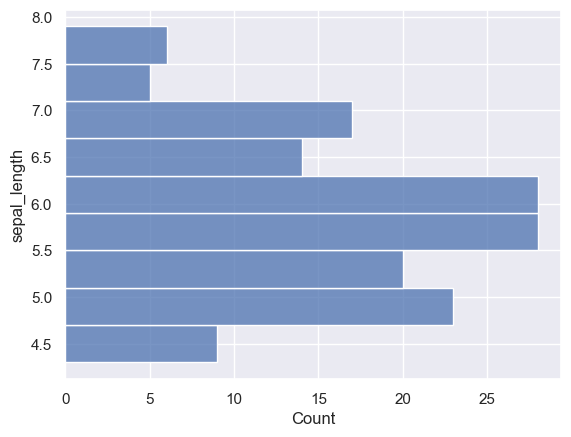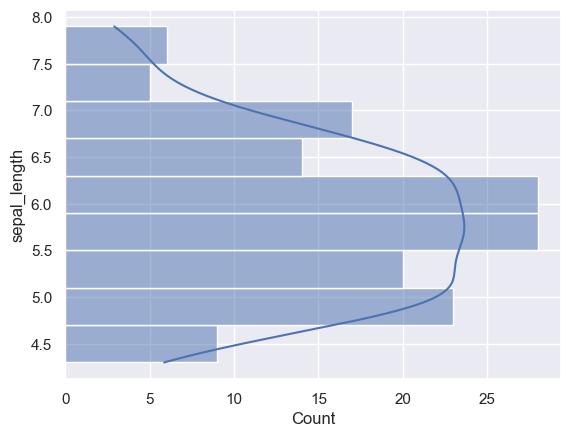## Libraries

First, we need to import the following libraries:

``````import seaborn as sns
import matplotlib.pyplot as plt``````

## Dataset

We'll use the iris dataset that is available using the `load_dataset()` function from seaborn

``df = sns.load_dataset("iris")``

## Flipped histogram

Flipping a histogram with seaborn is easy: you just have to specify that you want to display it on the `y axis`.

``````# set a grey background (use sns.set_theme() if seaborn version 0.11.0 or above)
sns.set(style="darkgrid")

# Create the plot
sns.histplot(data=df, y="sepal_length")
plt.show()``````## Flipped histogram with density estimation

If you want to add the density estimation, you have to add `kde=True` in the `histplot` function

``````# set a grey background (use sns.set_theme() if seaborn version 0.11.0 or above)
sns.set(style="darkgrid")

# Create the plot
sns.histplot(data=df, y="sepal_length", kde=True)
plt.show()``````## Going further

This post explains how to create a flipped histogram with seaborn.

You might be interested in creating histogram with multiple groups or controlling the bins.

## Contact & Edit

👋 This document is a work by Yan Holtz. You can contribute on github, send me a feedback on twitter or subscribe to the newsletter to know when new examples are published! 🔥